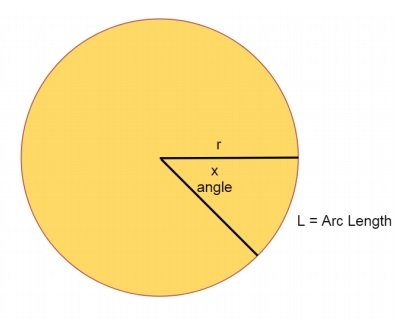# Arc length from given Angle?

Here we will see how to get the arc length from the given angle. One circle is given. The radius of the circle is given. Our task is to get the arc length using the radius and the angle. The angle is in degree.Here r and x is given. We have to find the value of L. The formula is like below −

𝐿 = 2𝜋𝑟 ∗ (𝑥/360)

## Example

#include <iostream>
using namespace std;
float getArcLength(float r, float x){
return (2 * 3.1415f * r) * (x / 360.0f);
}
int main() {
}
Arc Length: 9.4245